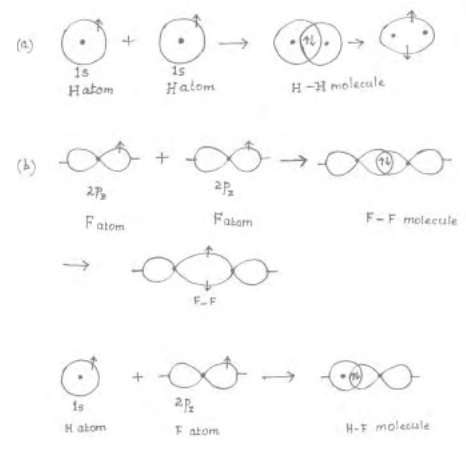Home | | Chemistry | Directional Properties of Covalent Bonds

# Directional Properties of Covalent BondsWhen the overlapping of orbitals occur along the internuclear axis (Line joining the two nuclei) then the electron orbitals merge to form cylindrically symmetrical region and bond is called as 'sig-ma' bond. In a 'sig-ma' bond, maximum extent overlap of orbitals are possible and the bond formed is also stronger.

Directional Properties of Covalent Bonds

When the overlapping of orbitals occur along the internuclear axis (Line joining the two nuclei) then the electron orbitals merge to form cylindrically symmetrical region and bond is called as 'sig-ma' bond. In a 'sig-ma' bond, maximum extent overlap of orbitals are possible and the bond formed is also stronger.

For e.g.: H-H bond is a 'sig-ma' bond

Consider the valence bond description of O2 molecule : the valence shell electron configuration of each O atom is 2s2 2px2 2py1 2pz1. It is conventional to take z axis as the inter nuclear axis or molecular axis. Along the molecular axis, overlap of 2Pz orbital of two O atoms occur with cylindrical symmetry thus forming a 'sig-ma' bond.

The remaining two 2py  orbitals of two O atoms cannot overlap to the full extent like a 'sig-ma' bond as they do not have cylindrical symmetry around the internuclear axis. Instead, 2py, orbitals overlap laterally (sideways) above and below the axis and share the pair of electrons. The bond formed by lateral overlap of p orbitals above and below the axis together is called a �  3L   ERQG. Since 2py orbitals are perpendicular to 2porbitals, Pi bond formed is perpendicular to the sig-ma bond. The bonding in oxygen molecule is represented as in Fig.There are two bonds in O2 molucule. One of which is a 'Sig-ma' bond and another is Pi bond.

Similarly, in N2 molecule, 3 bonds are present between 2N atoms. The nature of orbital overlaps in the 3 bonds can be considered as in fig. (b). to the 'sig-ma' bond. Thus bonding in oxygen molecule is represented as in Fig.(a).

There are two bonds in O2 molecule. One of which is a 'sig-ma' bond and another is Pi bond.

Similarly, in N2 molecule, 3 bonds are present between 2N atoms. The nature of orbital overlaps in the 3 bonds can be considered as in Fig.

The valence electronic configuration of nitrogen atom is 2s2 2px1 2py1 2pz1.Cylindrically symmetrical overlap of two 2pz orbitals a 'sig-ma' bond and lateral overlap of two 2py orbitals give a Pi bond perpendicular to 'sig-ma' bond. Similarly, two 2pz orbitals laterally overlap to giv another Pi bond which is perpendicular to both 'Sig-ma' , 'Pi' bonds.

Based on the valence bond orbital overlap theory, the H2O molecule is viewed to be formed by the overlap 1s orbital of a H atom with 2py orbital of O atom containing one electron each forming a 'Sig-ma' bond. Another  'Sig-ma' bond is also formed by the overlap of 1s orbital of another H atom with 2px orbital of O atom each containing an unpaired electron. The bond angle is therefore 90o (i.e : HOH bond angle is 90o), since 2px and 2py orbitals are mutually perpendicular to each other. However the actual bond angle value is found to be 104o. Therefore based on VB theory, pure orbital overlaps does not explain the geometry in H2O molecule.

Similarly, in NH3, according to VB theory each of N-H bonds are formed by the overlap of a 2p orbital of N and 1s orbital of H atoms respectively. Here again the bond angle of HNH bond is predicted as 90o. Since 2px, 2py and 2pz orbitals of N are mutually perpendicular. However the experimental bond angle of HNH bond value is found to be 107o.

Study Material, Lecturing Notes, Assignment, Reference, Wiki description explanation, brief detail
11th 12th std standard Class Organic Inorganic Physical Chemistry Higher secondary school College Notes : Directional Properties of Covalent Bonds |